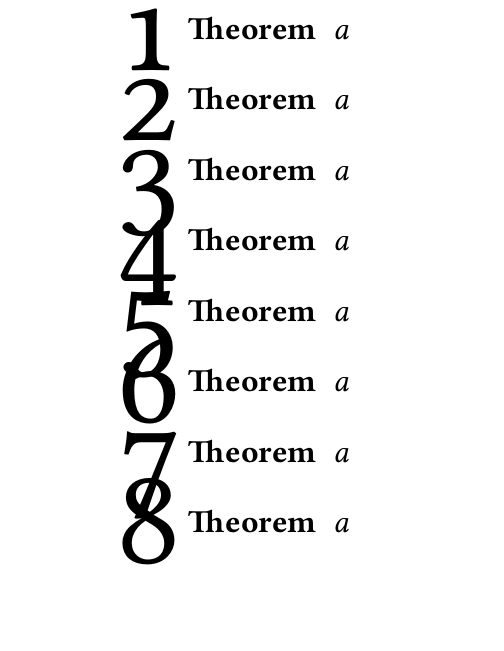Formatting theorems with drop-cap'd theorem numbers

I want to mark theorems with a big (drop cap) theorem number in the margin. I couldn't get LaTeX to raise the dropcap without using a smash, but then this causes numbers to overlap if theorems are short enough.

Is there a way to require extra padding around theorems?MWE:

\documentclass{article}
\usepackage{amsmath}
\usepackage[amsmath,thmmarks]{ntheorem}
\usepackage{lettrine}
\usepackage{libertine}
\newcommand{\numfont}{\normalfont\oldstylenums{\lettrine[lhang=1,lines=3,lraise=.5]{\smash{#1}}{}}}}
%modified code from the margin theorem style
\makeatletter
\newtheoremstyle{dropmargin}%
\makeatother
\theoremstyle{dropmargin}
\newtheorem{theorem}{Theorem}

\begin{document}
\begin{theorem} a \end{theorem}
\begin{theorem} a \end{theorem}

\begin{theorem} a \end{theorem}

\begin{theorem} a \end{theorem}

\begin{theorem} a \end{theorem}

\begin{theorem} a \end{theorem}

\begin{theorem} a \end{theorem}
\begin{theorem} a \end{theorem}

\end{document}


One option would be to use a \raisebox instead of \smash; using the two optional argument you can now have a better control of the height/depth you want TeX to see for your dropped numbers. You might want to adjust the settings to suit your needs:

\documentclass{article}
\usepackage{amsmath}
\usepackage[amsmath,thmmarks]{ntheorem}
\usepackage{lettrine}
\usepackage{libertine}
\newcommand{\numfont}{\normalfont\oldstylenums{\raisebox{10pt}[18pt][10pt]{\lettrine[lhang=1,lines=3]{#1}{}}}}
%modified code from the margin theorem style
\makeatletter
\newtheoremstyle{dropmargin}%
\makeatother
\theoremstyle{dropmargin}
\newtheorem{theorem}{Theorem}

\newcommand\Text{Some test text for illustration and here there are some more words test text for illustration and here there are some more words test text for illustration and here there are some more words test text for illustration and here there are some more words}

\begin{document}

\Text
\begin{theorem} a \end{theorem}
\Text
\begin{theorem} a \end{theorem}

\begin{theorem} a \end{theorem}

\begin{theorem} a \end{theorem}

\begin{theorem} a \end{theorem}

\begin{theorem} a \end{theorem}

\begin{theorem} a \end{theorem}

\begin{theorem} a \end{theorem}

\end{document}The solution by Gonzalo incudes problem: when theorem has more than one line than we see no equidistant lines. Another solution is to use \prevgraph in order to know how many lines in the theorem is. If this value is 1 or 2 then we add an additional vertical space. You can try this by adding following macro to your code (after your \newtheorem command):

\def\endtheorem{\@endtheorem \ifcase\prevgraf \or \bigskip \or \medskip\fi}